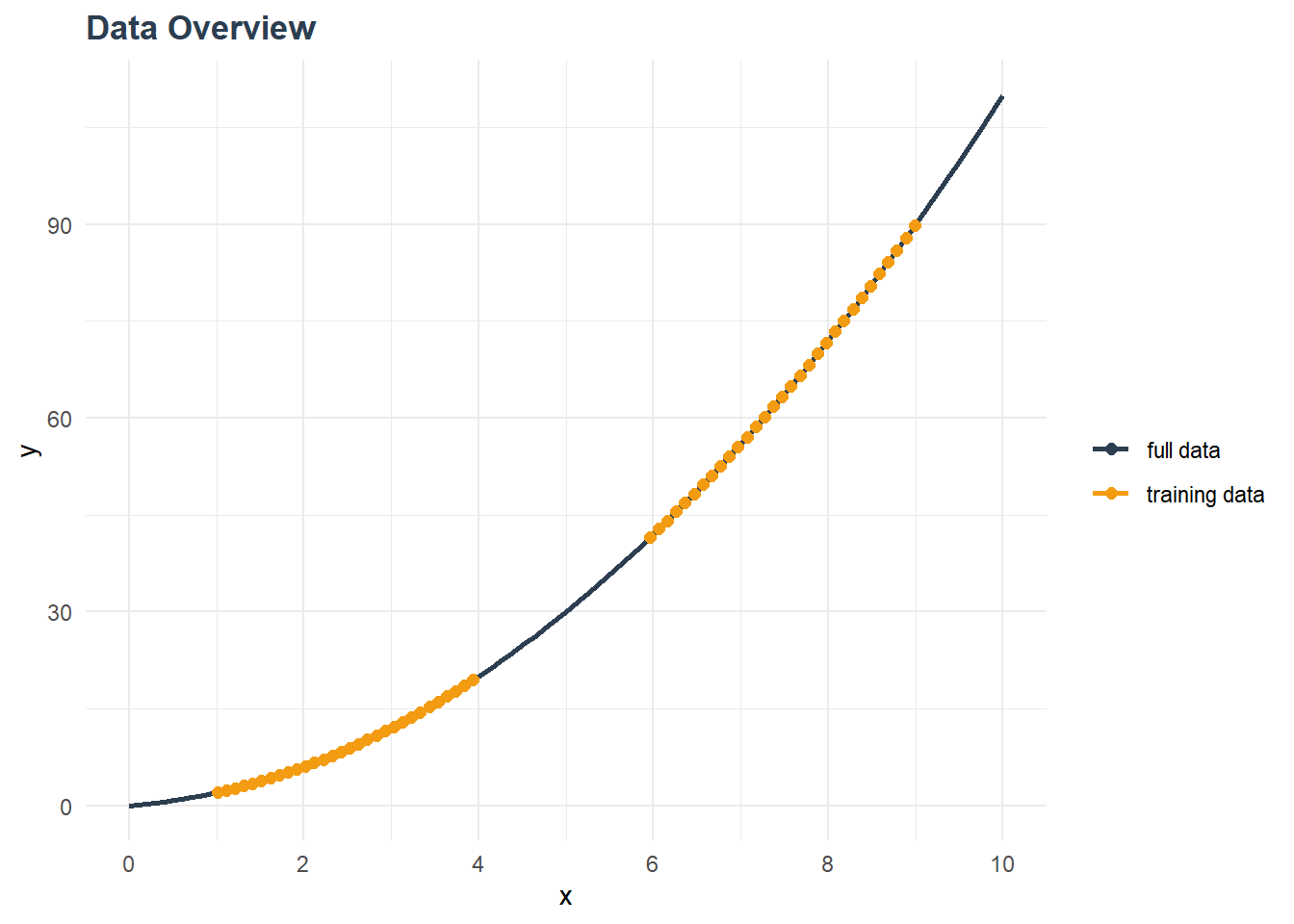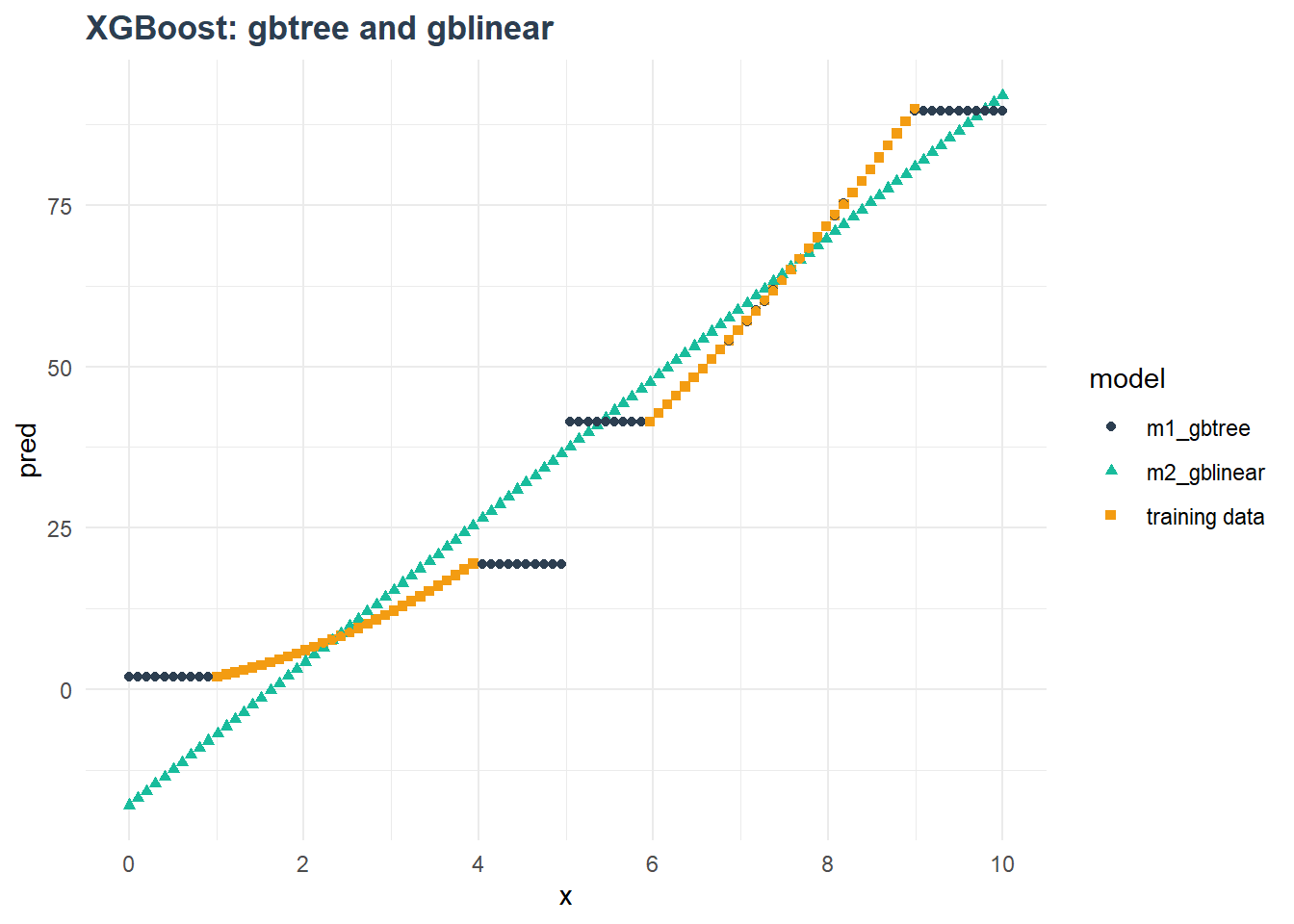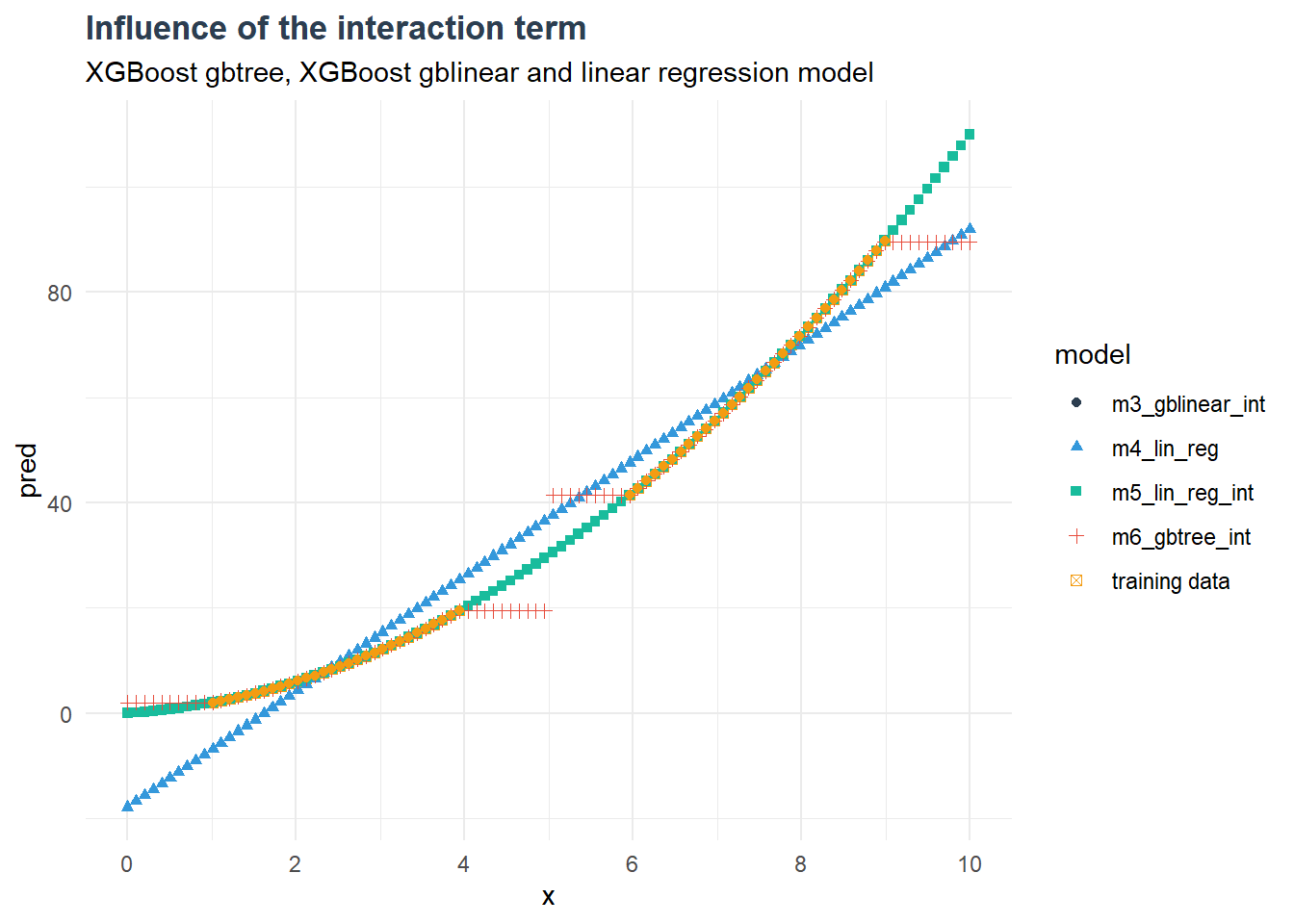Select Page

## Background

The XGBoost framework has become a very powerful and very popular tool in machine learning. This library contains a variety of algorithms, which usually come along with their own set of hyperparameters. This allows to combine many different tunes and flavors of these algorithms within one package. We can model various classification, regression or ranking tasks by using trees and linear functions, by applying different regularization schemes and by adjusting many other aspects of the individual algorithms.

These options are governed by hyperparameters. They can be separated into two classes: parameters that set a model’s characteristics and parameters that adjust a model’s behavior. An example for the first type is the model’s objective, where we set the type of the prediction variable. Obviously, a binary classification task will have a different output than a numerical prediction. The second set of parameters manages the training process. For example, the learning rate, usually called eta, adjusts the information gain for each learning step and thus prevents overfitting.

This article focuses on two specific parameters, that appear to be entangled and might cause some confusion: the objective and the booster.

For more information on the full set of parameters see the official XGBoost documentation.

## Short overview of two XGBoost parameters: booster and objective

• The booster parameter sets the type of learner. Usually this is either a tree or a linear function. In the case of trees, the model will consist of an ensemble of trees. For the linear booster, it will be a weighted sum of linear functions.
• The objective determines the learning task, thus the type of the target variable. The available options include regression, logistic regression, binary and multi classification or rank. This option allows to apply XGBoost models to several different types of use cases. The default value is “reg:squarederror” (previously called “reg:linear” which was confusing and was therefore renamed (see details)).

It should be noted, that the objective is independent of the booster. Decision trees are not only able to perform classification tasks but also to predict continuous variables with a certain granularity for the data input range used in the training.

Thus, the objective is always determined by the modeling task at hand, while the two common booster choices can be valid for the same problem.

# Visualizing different boosters

To illustrate the differences between the two main XGBoost booster tunes, a simple example will be given, where the linear and the tree tune will be used for a regression task. The analysis is done in R with the “xgboost” library for R.

In this example, a continuous target variable will be predicted. Thus, the correct objective is “reg:squarederror”. The two main booster options, gbtree and gblinear, will be compared.

The dataset is designed to be simple. The input parameter x is a continuous variable, ranging from 0 to 10. No noise is added to keep the task easy. The target variable is generated from the input parameter:

y=x+

The training data is chosen to be a subset of the full dataset, by selecting two subranges, [1:4] and [6:9]. This is illustrated in the figure below (yellow data points). By this, it can be tested how well the model behaves on unseen data.With this training data, two XGBoost models are generated, m1_gbtree with the gbtree booster and m2_gblinear with the gblinear booster. The trained models are then used to generate predictions for the whole data set.model
RMSE (full data)
MAE (full data)
RMSE (train data)
MAE (train data)
m1_gbtree 4.91 2.35 0.05 0.03
m2_gblinear 7.74 6.39 4.50 3.89

The predictions for the full dataset are shown in the plot above along with the full dataset. The first model, which uses trees, predicts the training data well in those regions, where the model was supplied with training data. However, in the outer regions (x<1  and x>9) as well as in the central region (4<x<6) discrepancies arise. The tree-based model replicates the prediction of the closest known datapoint, thus generating horizontal lines. This is always the case when trees are used for continuous predictions. No formula is learned which allows for inter- or extrapolation.

The second model uses a linear function for each learner in the gradient boosting process. The weighted combination of these learners is still a linear function. This explains the model’s behavior: The predictions follow a linear curve rather than the non-linear behavior of the data.

When looking at the metrics for the full dataset, the tree-based model shows a lower RMSE (4.9 versus 7.7) and MAE (2.4 versus 6.4) than the linear model. It should be noted, that the other hyperparameter of the models were not tuned and as a result, these numbers do not necessarily reflect the optimum. Nevertheless, they show how poor the models perform on the full dataset. The metrics that only consider training data reflect the differences in the modeling. The tree-based model represents the training data well, while the linear model does not. This is due to the fact, that the dependency of the target variable with the input variable is non-linear.

Can the models be improved, if a non-linear variable is supplied? As a test, each model is trained on modified input data, which is based on the original input variable as well as a new variable x int =x² .

The new variable contains the interaction term, which causes the non-linear behavior in the first place.

Additionally, a simple linear regression model is added for comparison, with and without interaction term.model
RMSE (full data)
MAE (full data)
RMSE (train data)

m1_gbtree 4.91 2.35 0.05
m6_gbtree_int 4.91 2.35 0.05
m2_gblinear 7.74 6.39 4.50
m3_gblinear_int 0.00 0.00 0.00
m4_lin_reg 7.74 6.39 4.50
m5_lin_reg_int 0.00 0.00 0.00

From this we can learn a few things:

Firstly, the interaction term significantly improves the linear models. They show perfect agreement with the full dataset. Here, the regression function exactly models the true relation between input and target variables. In addition, this trained function allows to extrapolate well to unseen data.

Secondly, the tree based model did not improve by including the interaction term. This can be explained by considering again how trees work for a regression task. A tree splits the input space of the training data into fine categories, which are represented by its leaves. The prediction value for each leaf is learned from the target variable, thus the target variable is discretized. Adding more input variables refines the splitting of the input space. In this example, the original input variable x is sufficient to generate a good splitting of the input space and no further information is gained by adding the new input variable.

Finally, the linear booster of the XGBoost family shows the same behavior as a standard linear regression, with and without interaction term. This might not come as a surprise, since both models optimize a loss function for a linear regression, that is reducing the squared error. Both models should converge to the optimal result, which should be identical (though maybe not in every last digit). This comparison is of course only valid when using the objective “reg:squarederror” for the XGBoost model.

# Summary

In this article, the two main boosters gblinear and gbtree of the XGBoost family were tested with non-linear and non-continuous data. Both boosters showed conceptual limits regarding their ability to extrapolate or handle non-linearity. Tree-based models allow to represent all types of non-linear data well, since no formula is needed which describes the relation between target and input variables. This is an enormous advantage if these relations and interactions are unknown. Linear models on the other hand cannot learn other relations than pure linear ones. If these additional interactions can be supplied, linear models become quite powerful.

The second aspect considered the fact that the training data does not always cover the full data range of the use case. Here, the model needs to inter- or extrapolate from known datapoints to the new regions. In the case of trees, no formula is available, which would allow to navigate these areas and provide meaningful prediction values. In contrast, this is the main advantage of linear regression models – if the same assumptions can be applied to the new data. In other words, if the new data behaves in the same way.

Do you have any question? Simply email to: marketing@avato.net

Imprint:
Date: December 2019
Author: Verena Baussenwein
Contact: marketing@avato.net
www.avato-consulting.com